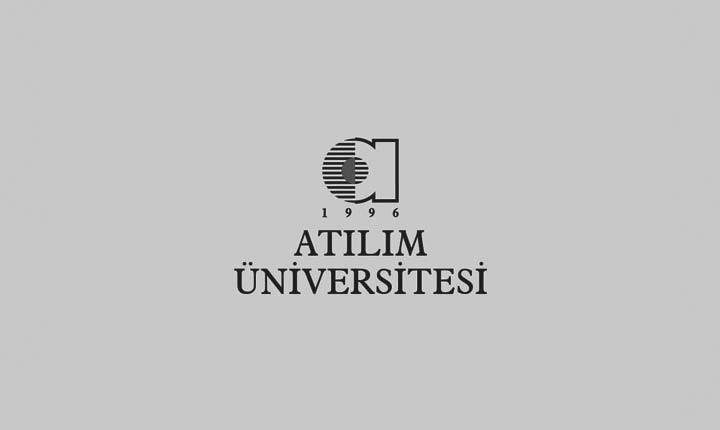#### Prof. Dr. Ayhan AYDIN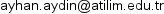/ +90 (312) 586 8233

Bachelor's, Master's, PhD: Middle East Technical University

Research Interests : Numerical Solution of Partial Differential Equations, Geometric Methods, Numerical Solutions of Ordinary Differential Equations, Nonstandard finite difference schemes.

Detailed CV#### Prof. Dr. Tanıl ERGENÇ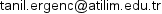/ +90 (312) 586 8645

Bachelor's, Master's, PhD: Middle East Technical University

Research Interests : Numerical Solution of Nonlinear Algebraic Equations, Impulsive Differential Equations, Dynamical Systems, Numerical Solutions of Ordinary Differential Equations, Ordinary Differential Equations, Numerical Analaysis, Numerical Solution of Systems of Linear Equations, Ordinary Differential Equations, Numerical Solutions of Ordinary Differential Equations, Initial Value Problems, Dynamical Systems, Numerical Solution of Partial Differential Equations, Numerical Solution of Nonlinear Algebraic Equations, Numerical Analaysis.

Detailed CV#### Prof. Dr. İnci ERHAN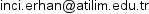/ +90 (312) 586 8222

Bachelor's, Master's: Marmara University PhD: Middle East Technical University

Research Interests : Fixed Point Theory, Numerical Analaysis, Numerical Solutions of Ordinary Differential Equations, Ordinary Differential Equations.

Detailed CV#### Prof. Dr. Sofiya OSTROVSKA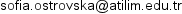/ +90 (312) 586 8211

Bachelor's, Master's: Kharkov State University PhD: Kiev State University

Research Interests : Probability Theory, Approximation Theory.

Detailed CV#### Prof. Dr. Abdullah ÖZBEKLER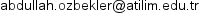/ +90 (312) 586 8670

Bachelor's, Master's, PhD: Middle East Technical University

Research Interests : Time Scale Calculus, Partial Differential Equations, Ordinary Differential Equations, Impulsive Differential Equations, Functional Differential Equations, Dynamical Systems, Difference Equations, Differential Inequalities.

Detailed CV#### Prof. Dr. Uğur YÜKSEL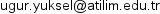/ +90 (312) 586 8225

Bachelor's, Master's, PhD: Ankara University

Research Interests : Theory of Associated Operators in the Sense of W. Tutschke, Quaternionic Analysis, Pseudo-Analytic Functions, Partial Differential Equations, Ordinary Differential Equations, Initial Value Problems, Generalized Analytic Functions, Fixed Point Theory, Complex Boundary Value Problems for Partial Differential Equations, Clifford Analysis.

Detailed CV#### Assoc. Prof. Dr. Rezan SEVİNİK ADIGÜZEL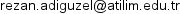/ +90 (312) 586 8240

Bachelor's: Ankara University Master's, PhD: Middle East Technical University

Research Interests : Special Functions, Classical Orthogonal Polynomials, Ordinary Differential Equations, Numerical Solutions of Ordinary Differential Equations.

Detailed CV#### Assoc. Prof. Dr. Ümit AKSOY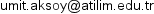/ +90 (312) 586 8234

Bachelor's, Master's, PhD: Middle East Technical University

Research Interests : Partial Differential Equations, Generalized Analytic Functions, Complex Boundary Value Problems for Partial Differential Equations, Mathematical Finance.

Detailed CV#### Assoc. Prof. Dr. Ferihe ATALAN OZAN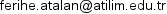/ +90 (312) 586 8659

Bachelor's, Master's: Ege University PhD: Middle East Technical University

Research Interests : Geometric Topology.

Detailed CV#### Assoc. Prof. Dr. Mehmet TURAN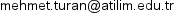/ +90 (312) 586 8665

Bachelor's, PhD: Middle East Technical University

Research Interests : Time Scale Calculus, Impulsive Differential Equations, Functional Differential Equations, Dynamical Systems, Ordinary Differential Equations, Theory of Bifurcation, Center Manifold.

Detailed CV#### Assoc. Prof. Dr. Burcu GÜLMEZ TEMÜR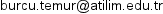/ +90 (312) 586 8226

Bachelor's, Master's, PhD: Middle East Technical University

Research Interests : Cryptology, Coding Theory, Algebraic Function Fields, Algebraic Curves.

Detailed CV#### Assoc. Prof. Dr. Fatih SULAK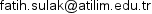/ +90 (312) 586 8290

Bachelor's, Bachelor's, Master's, PhD: Middle East Technical University

Research Interests : Cryptology.

Detailed CV